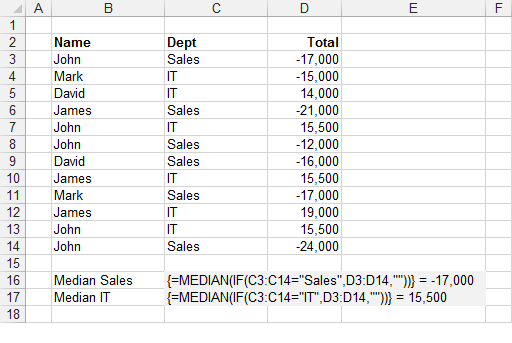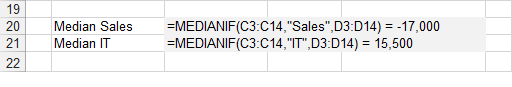### MEDIANIF

Returns the median value in a range of values that match a certain condition.

`Option Base 1 'rgeCriteria - The range of cells containing the criteria you want to check.'sCriteria - The criteria value you want to match.'rgeMedianRange - The range of corresponding values you want the median of.Public Function MEDIANIF(ByVal rgeCriteria As Range, _                          ByVal sCriteria As String, _                          ByVal rgeMedianRange As Range) As Single           Dim iconditioncolno As Integer Dim inumberscolno As Integer Dim lrowno As Long Dim lmatch As Long Dim arsngvalues() As Single Dim sngmedian As Single Dim bsorted As Boolean Dim vcellvalue As Variant    iconditioncolno = rgeCriteria.Column    inumberscolno = rgeMedianRange.Column    ReDim arsngvalues(rgeCriteria.Rows.Count)       For lrowno = 1 To rgeCriteria.Rows.Count       vcellvalue = rgeCriteria.Parent.Cells(rgeCriteria.Row + lrowno - 1, inumberscolno).Value       If rgeCriteria.Parent.Cells(rgeCriteria.Row + lrowno - 1, iconditioncolno).Value = sCriteria And _       IsNumeric(vcellvalue) = True Then          lmatch = lmatch + 1          arsngvalues(lmatch) = rgeCriteria.Parent.Cells(rgeCriteria.Row + lrowno - 1, inumberscolno).Value       End If       If lmatch > 0 And IsEmpty(vcellvalue) = True Then Exit For    Next lrowno    ReDim Preserve arsngvalues(lmatch)    Do       bsorted = True       For lrowno = 2 To lmatch          If arsngvalues(lrowno - 1) > arsngvalues(lrowno) Then             sngmedian = arsngvalues(lrowno - 1)             arsngvalues(lrowno - 1) = arsngvalues(lrowno)             arsngvalues(lrowno) = sngmedian             bsorted = False          End If       Next lrowno    Loop Until bsorted = True        If lmatch Mod 2 = 0 Then MEDIANIF = (arsngvalues(CInt(lmatch / 2)) + arsngvalues(1 + CInt(lmatch / 2))) / 2    If lmatch Mod 2 = 1 Then MEDIANIF = arsngvalues((lmatch + 1) / 2) End Function `

There is an Array Formula equivalent if you do not want to use the user defined function (see below).
This includes hidden rows.
This user defined function is similar to the built-in SUMIF function.#### Array Formula

To find out more about Array Formulas please refer to the Array Formulas section.
To find out more about the individual functions please refer to the MEDIAN function and IF function pages.
This example illustrates how to use an array formula to obtain the median value in a column based on a condition.The formula in row 16 returns the median in the "Sales" department.
The formula in row 17 returns the median in the "IT" department.
The formula [C3:C14="Sales"] returns an array of 1's and 0's indicating if the value in column "C" satisfies the condition.
Every number 1 in this array represents the value True in the IF statement and therefore has its corresponding value returned from column "D".
Every number 0 in this array represents the value False in the IF statement and therefore an empty string is returned in this case. The MEDIAN function will ignore any string values.
The use of the empty string is to ensure that the formula works with both positive and negative numbers.
This array of numbers is then passed to the MEDIAN() function to obtain the largest number.
Remember that you must press (Ctrl + Shift + Enter) to enter the formulas.

#### Important

You cannot use column references (such as "A:A" or "D:D") in your array formulas.
Any cell ranges passed to an array formula must have the same number of rows. This is to ensure that all the temporary arrays used are the same length.